# Precalculus : Limits

## Example Questions

1 3 Next →

### Example Question #21 : Limits

Find the limit of the function: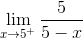Possible Answers: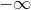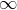The limit can't be determined.

Correct answer:Explanation:

Substituting the value ofwill yield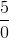, which is not in indeterminate form.  Therefore, L'Hopital's rule cannot be used.

The question asks for the limit as x approaches to five from the right side in the graph. As the graph approaches closer and closer to, the y-value decreases to negative infinity and will never touch.

The correct answer is:### Example Question #22 : Limits

Find the limit.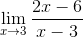Possible Answers: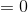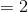Does not exist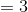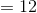Correct answer:Explanation:

In the unsimplified form, the limit does not exist; however, the numerator can be factored and simplified.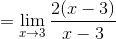### Example Question #23 : Limits

Find the limit: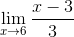Possible Answers: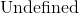Correct answer:Explanation:

The first and only step is to substitute the value ofinto the function.  Since there is not a zero denominator or an indeterminate form, we do not have to worry about L'Hopital or an undefined limit.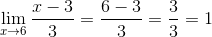The limit will approach to.

### Example Question #24 : Limits

Find the following limit: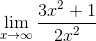Possible Answers: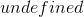Correct answer:Explanation:

To solve, simply realize you are dealing with a limit whose numerator and demominator have the same max power. Thus the limit is simply the division of their coefficient.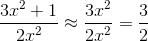### Example Question #1 : Determine If A Function Is Continuous Using Limits

Find the domain where the following function is continuous: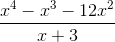Possible Answers: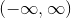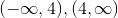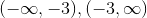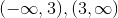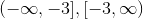Correct answer:Explanation:

The function in the numerator factors to: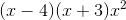so if we cancel the x+3 in the numerator and denominator we have the same function but it is continuous. The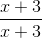gives us a hole at x=-3 so our function is not continuous at x=-3.

### Example Question #2 : Determine If A Function Is Continuous Using Limits

What are the discontinuities in the following function and what are their types?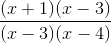Possible Answers: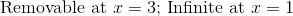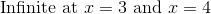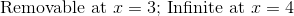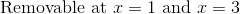Correct answer:Explanation:

Since the factor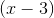is in the numerator and the denominator, there is a removable discontinuity at.  The function is not defined at, but function would move towards the same point for the resultant function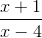.

Since the factor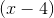cannot be factored out, there is an infinite discontinuity at.  The denominator will get very small and the numerator will move toward a fixed value.

There is no discontinuity at all at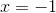.  The function simply evaluates to zero at this point.

### Example Question #3 : Determine If A Function Is Continuous Using Limits

Determine if the function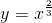is continuous atusing limits.

Possible Answers:

No, it is not continuous because the lefthand limit does not match the righthand limit.

Yes, it is continuous because the lefthand and righthand limits are equal.

No, it is not continuous because the lefthand and righthand limits do not equal the value of the function at 0.

Yes, it is continuous because the righthand and lefthand limits are equal to the actual value of the function.

Correct answer:

Yes, it is continuous because the righthand and lefthand limits are equal to the actual value of the function.

Explanation:

In order to determine if a function is continuous at a point three things must happen.

1) Taking the limit from the lefthand side of the function towards a specific point exists.

2) Taking the limit from the righthand side of the function towards a specific point exists.

3) The limits from 1) and 2) are equal and equal the value of the original function at the specific point in question.

In our case,

1)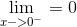2)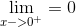3)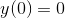Because all of these conditions are met, the function is continuous at 0.

### Example Question #4 : Determine If A Function Is Continuous Using Limits

Determine if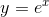is continuous on all points of its domain.

Possible Answers:

Yes, it is continuous because the righthand and lefthand limits are equal to the actual values of the function.

Yes, it is continuous because the lefthand and righthand limits are equal.

No, it is not continuous because the lefthand limit does not match the righthand limit.

No, it is not continuous because the lefthand and righthand limits do not equal the value of the function at 0.

Correct answer:

Yes, it is continuous because the righthand and lefthand limits are equal to the actual values of the function.

Explanation:

First, find that at any point where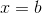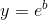.

Then find that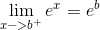and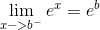.

As these are all equal, it can be determined that the function is continuous on all points of its domain.

### Example Question #5 : Determine If A Function Is Continuous Using Limits

Let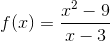. Determine if the function is continuous using limits.

Possible Answers: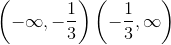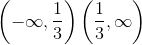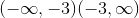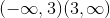Correct answer:Explanation:

Asapproaches, the functionapproaches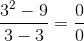, which is undefined. However, if we factor, we get: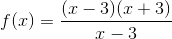Thefactors in the numerator and denominator cancel out, leaving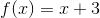.

Therefore, our function is continuous at all values offrom.

1 3 Next →

### All Precalculus Resources